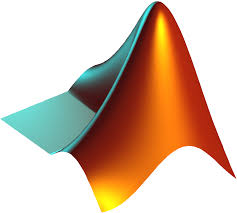#### Resources

Below are links to various resources for the course.

##### Documents
###### Lecture notes

 Lecture 4 Lecture 5 Lecture 6 Lecture 7 Lecture 8 and 9 Lecture 10 Lecture 11 Lecture 12 Lecture 13 Lecture 14 Lecture 15 Lecture 16 Lecture 17 Lecture 18 Lecture 19 Lecture 20 Lecture 21 Lecture 22 Lecture 23 Lecture 24 Lecture 25 Lecture 26 Lecture 26, Lemma 8 (proof)

###### Fibonacci sequence

Fibonacci numbers
Golden ratio
Vi Hart - Fibonacci sequence in plants - part I, part II, part III

##### Wolfram Alpha
Wolfram Alpha is an online answer engine that is very useful for mathematical problems. It can differentiate and integrate, plot functions and much more.Wolfram Alpha

##### Maple
Like Wolfram Alpha, Maple can be used for checking differentiation, plotting functions or visualizing surfaces in three-space. At Dartmouth there is licensed software available for students. If you would like to install Maple you can follow the instructions here:Maple

Below is a Quickstart Guide which lists various things you can do with Maple giving both the mathematical expression familiar to you as well as the syntactically correct command in Maple. To download the two worksheets (on integration and graphing) save the two files.
##### MATLAB

MATLAB, a contraction of "matrix laboratory", is a computing environment and programming language developed by MathWorks. It can be used in a similar fashion as Maple. Dartmouth has a site license for MATLAB. You can download it here:MATLAB

Bjoern Muetzel
Last updated March 07, 2018